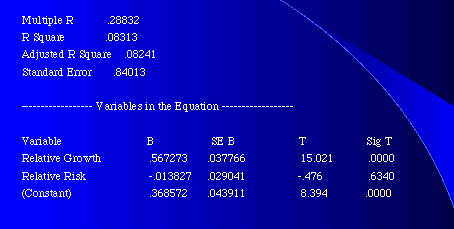Relative PE Ratios
• The relative PE ratio of a firm is the ratio of the PE of the firm to the PE of the market.

Relative PE = PE of Firm / PE of Market

• While the PE can be defined in terms of current earnings, trailing earnings or forward earnings, consistency requires that it be estimated using the same measure of earnings for both the firm and the market.
• Relative PE ratios are usually compared over time. Thus, a firm or sector which has historically traded at half the market PE (Relative PE = 0.5) is considered over valued if it is trading at a relative PE of 0.7.

Relative PE: Cross Sectional Distribution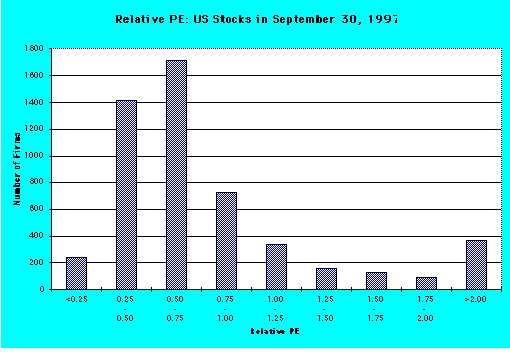Relative PE: Distributional Statistics

Mean 1.00 Std Dev .997

Variance .995 Kurtosis 15.289

S.E. Kurt .068 Skewness 3.713

S.E. Skew .034 Minimum .00

Maximum 6.27 Median 0.60

Relative PE: Determinants

To analyze the determinants of the relative PE ratios, let us revisit the discounted cash flow model we developed for the PE ratio. Using the 2-stage DDM model as our basis (replacing the payout ratio with the FCFE/Earnings Ratio, if necessary), we get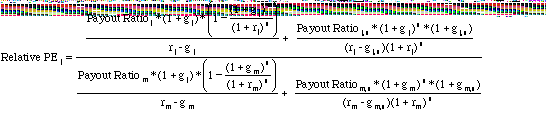where Payoutj, gj, rj = Payout, growth and risk of the firm

Payoutm, gm, rm = Payout, growth and risk of the market

Relative PE: A Simple Example

Consider the following example of a firm growing at twice the rate as the market, while having the same growth and risk characteristics of the market:

Firm Market

Expected growth rate 20% 10%

Length of Growth Period 5 years 5 years

Payout Ratio: first 5 yrs 30% 30%

Growth Rate after yr 5 6% 6%

Payout Ratio after yr 5 50% 50%

Beta 1.00 1.00

Riskfree Rate = 6%

Estimating Relative PE

The relative PE ratio for this firm can be estimated in two steps. First, we compute the PE ratio for the firm and the market separately: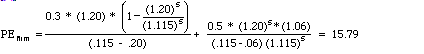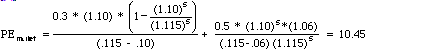Relative PE Ratio = 15.79/10.45 = 1.51

Relative PE and Relative Growth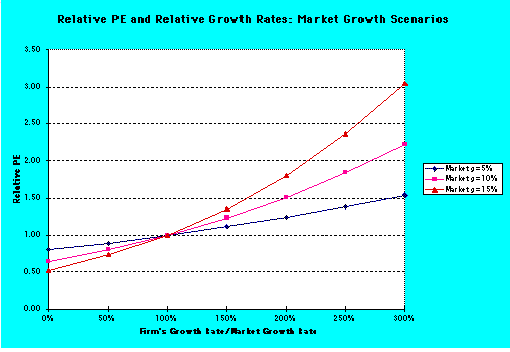Relative PE: Another Example

In this example, consider a firm with twice the risk as the market, while having the same growth and payout characteristics as the firm:

Firm Market

Expected growth rate 10% 10%

Length of Growth Period 5 years 5 years

Payout Ratio: first 5 yrs 30% 30%

Growth Rate after yr 5 6% 6%

Payout Ratio after yr 5 50% 50%

Beta in first 5 years 2.00 1.00

Beta after year 5 1.00 1.00

Riskfree Rate = 6%

Estimating Relative PE

The relative PE ratio for this firm can be estimated in two steps. First, we compute the PE ratio for the firm and the market separately: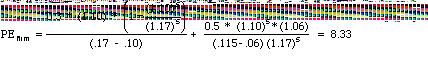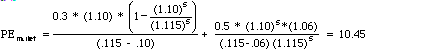• Relative PE Ratio = 8.33/10.45 = 0.80

Relative PE and Relative Risk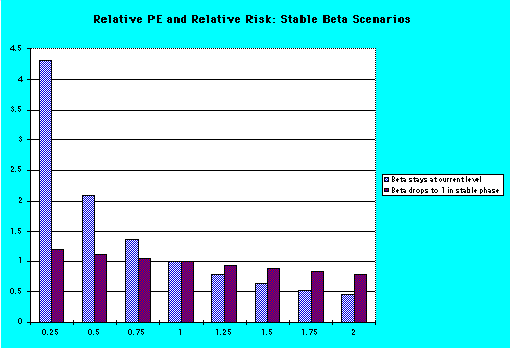Relative PE: Summary of Determinants

• The relative PE ratio of a firm is determined by two variables. In particular, it will
• increase as the firmís growth rate relative to the market increases. The rate of change in the relative PE will itself be a function of the market growth rate, with much greater changes when the market growth rate is higher. In other words, a firm or sector with a growth rate twice that of the market will have a much higher relative PE when the market growth rate is 10% than when it is 5%.
• decrease as the firmís risk relative to the market increases. The extent of the decrease depends upon how long the firm is expected to stay at this level of relative risk. If the different is permanent, the effect is much greater.
• Relative PE ratios seem to be unaffected by the level of rates, which might give them a decided advantage over PE ratios.

Relative PE Ratios: The Auto Sector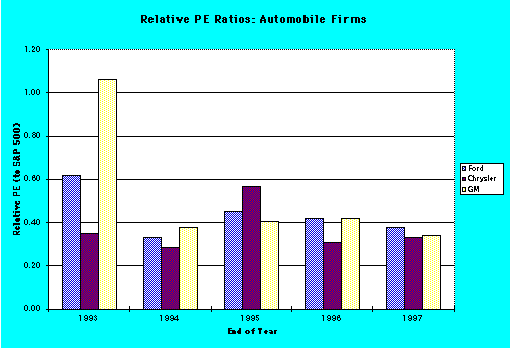Using Relative PE ratios

On a relative PE basis, all of the automobile stocks look cheap because they are trading at their lowest relative PE ratios in five years. Why might the relative PE ratio be lower today than it was 5 years ago?

Relative PE: Why do they change?

Historically, GM has traded at the highest relative PE ratio of the three auto companies, and Chrysler has traded at the lowest. In the last two or three years, this historical relationship has been upended with Ford now trading at the highest relative PE ratio. Analyst projections for earnings growth at the three companies are about the same. How would you explain the shift?

Relative PE Ratios: Market Analysis